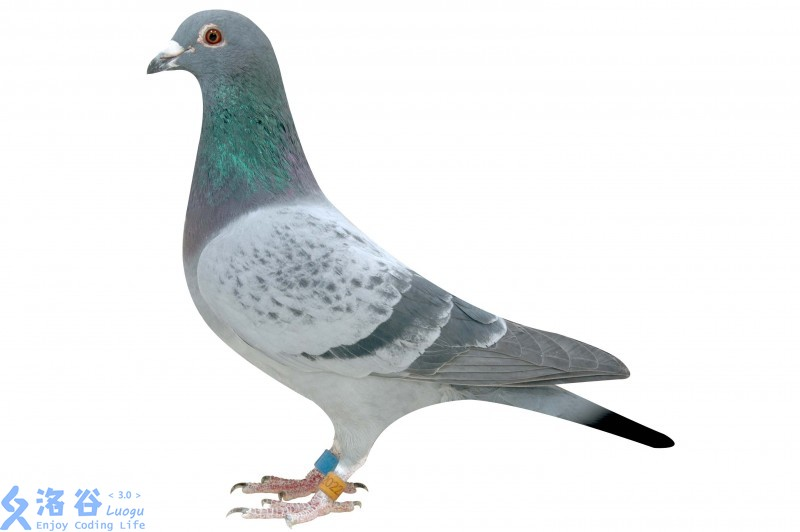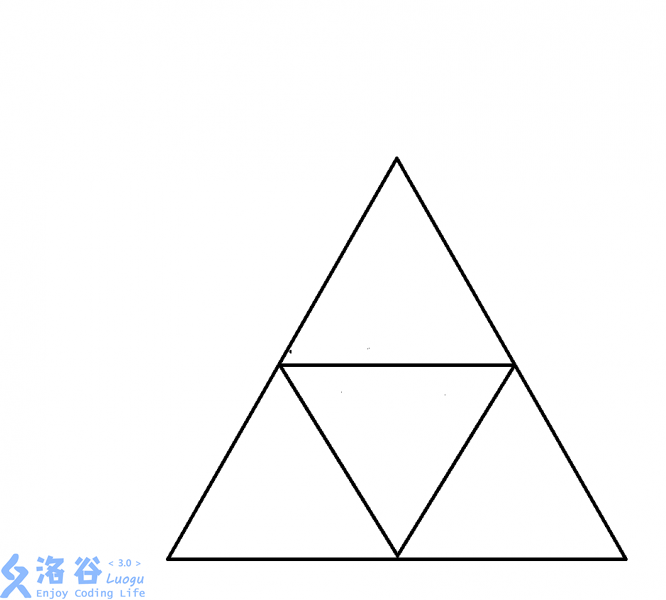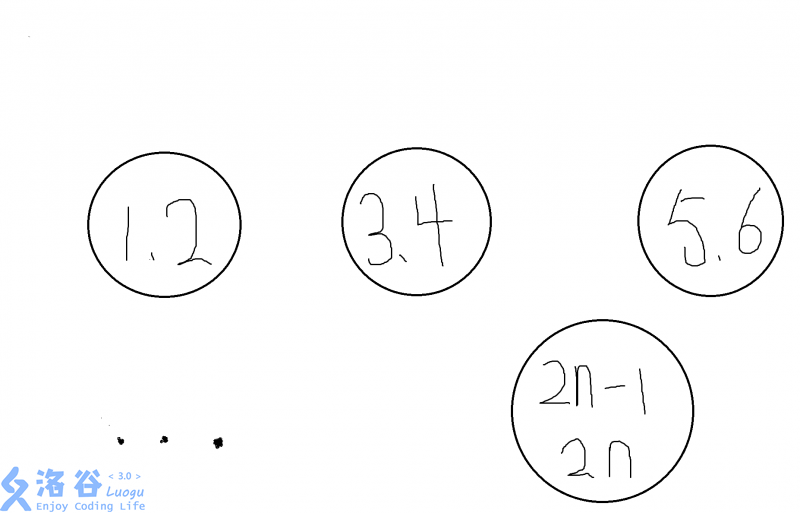# 第一部分：鸽巢原理a数组：but 我也很重要

＄：我好像也出现不少次• $a_1,a_2,a_3...a_m$

• 为正整数。

• $if$ 我们将

• $a_1+a_2+a_3+...+a_n-n+1$

• 个鸽子放入 $n$个笼子里， $then$，

||第一个笼子至少有 $a_1$只鸽子||第二个笼子至少有 $a_2$只鸽子||第三个笼子至少有 $a_3$只鸽子||...||第 $m$个笼子至少有 $a_m$只鸽子

# 鸽巢原理的应用

1.我们可以令 $a_1$表示第一天所刷的题数， $a_2$表示前两天所刷的题数， $a_3$表示前三天所刷的题数.之后以此类推

2.而题目说，由于每天都要至少刷1题，所以数列

• $a_1,a_2,a_3,a_4,...,a_{84}$

• 严格递增。另有 $a_1>=1$.又每周最多刷13题，故 $a_{84}<=13*12=156$.

3.因此又有：

• $1<=a_1<a_2<a_3<...<a_{84}<=156$.

4.同理，

• $a_1+11,a_2+11,a_3+11,...,a_{84}+11$

• 同样是一个严格递增序列。范围：

• $12<=a_1+11<a_2+11<a_3+11<...<a_{84}+11<=167$

5.我们把两个序列合起来看：

• $a_1,a_2,a_3,...,a_{84},a_1+11,a_2+11,a_3+11,...,a_{84}+11$

• 一共 $168$个数。其中每一个数都是 $1$到 $167$之间的一个整数。

6.根据鸽巢原理可得，其中必有两个数相等！！！

7.既然

• $a_1,a_2,a_3,...,a_{84}$

• 中必然无相等的两个数，

• 那么 $a_1+11,a_2+11,a_3+11,...,a_{84}+11$

• 中同理。那么，必然存在一个 $x$和一个 $y$,使得

• $a_x=a_y+11$;

8.从而得出结论：这个 $oier$在第

• $y+1,y+2,y+3,...,x$

• 天内一共刷了 $11$道题

# 应用二1.我们先画出一个边长为 $2$的等边三角形。

2.然后把三条边中点两两相连。就形成了这张图。

3.那么根据鸽巢原理，必然有两个点在一个边长为 $1$的小三角形里。

4.而我们知道，边长为 $1$的等边三角形里处处距离都小于等于 $1$

5.于是问题就解决了

# 应用三

1.要证明这个问题，我们就要利用一个互质的特性：两个相邻整数互质。

2.有了这个突破口，于是我们可以构造n个鸽巢，每一个里依次放入

• $1,2,3,...,2n$

• 这2n个数中的两个数。3.也就是说，我们要在这其中取出 $n+1$个数。

4.根据鸽巢原理，无论如何，我们都会抽空一个鸽巢。

5.一个鸽巢中的两个数肯定互质，所以问题就解决了。

【NOIP2010 提高组】记 $T$为一队列，初始时为空，现有n个总和不超过 $32$的正整数依次入队，如果无论这些数具体为什么值，都能找到一种出队的方式，使得存在某个时刻队列 $T$中的数之和恰好为 $9$，那么 $n$的最小值是___

1.第一眼看到此题，蒟蒻就知道自己只能根据结果推过程

2.刚开始看了一眼答案： $18$.

3.于是就根据这个开始推导过程。我们可以令 $a_i$表示前 $i$个数的和，并约定： $a_0=0$.

4.题目要求求出最小的 $n$，使得存在 $0<=x<y<=n$满足 $a_y=a_x+9$;

5.于是我们可将 $a$数组看做鸽子，用不能同时取的一组（差为 $9$）的集合构造笼子，

6.构造方法如下：一共有 $n=18$个集合按此方式选取：

• ${0,9}、{1,10}、{2,11}、...、{8,17}、{18,27}、{19,28}、{20,29}、...、{23,32}、{24}、{25}、{26}$。

7.由题意可知，我们一旦在某个集合中取了两个元素， $then$ 一定存在某个时刻队列 $T$中数的总和恰好为 $9$.

8.于是由鸽巢原理，我们可以得知： $n=18$一定满足条件.

1.我们还要证明一下 $n=17$不可行。

2.然鹅我们只需要举出反例即可：

• 1 1 1 1 1 1 1 1 10 1 1 1 1 1 1 1 1

3.说明：因为每到了 $8$个 $1$就被 $10$隔断，故不可行。

# 第二部分：Ramsey定理

• 此定理有一个广为流传的例子：6 个人中至少存在3人相互认识或者相互不认识。

• 转换：该定理等价于证明这6个顶点的完全图的边，用红、蓝二色任意着色，必然至少存在一个红色边三角形，或蓝色边三角形

2、设：如果两个人认识，则设这两个人组成的线段为红色；如果两个人不认识，则设这两个人组成的线段为蓝色。